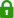# Texas Instruments TI-84 Plus CE Graphing Calculator

SKU: TEXTI84PLUSCE
Price:
Sale price \$133.49
Stock:
Sold out

####100% Secure Payments

• Graphing Calculator
• 1 Each
• Black
• Calculator features
Backlit Display, Clock, Impact Resistant Cover
Color
Black
Country of origin
Philippines
Manufacturer part number
TI84PLUSCE
Operations/ functionality
Add images to graph background, 15 colors for function graphing, Change style and color of axes and grids on graphs, Catalog Syntax Help, Alphabetical CATALOG of all TI calculator operations in one menu, Advanced functions accessed through pull-down display menus, Real and complex numbers calculated to 14-digit accuracy and displayed with 10 digits plus a 2-digit exponent, Graphs 10 rectangular functions, 6 parametric expressions, 6 polar expressions, and 3 recursively-defined sequences, Up to 10 graphing functions defined, saved, graphed and analyzed at one time, Sequence graphing mode shows time series plot, cobweb/stair-step plot and phase plots, User-defined list names. Lists store up to 999 elements, 17 interactive zoom features, Numeric evaluations given in table format for all graphing modes, Interactive analysis of function values, roots, maximums, minimums, integrals and derivatives, 8 different graph styles for differentiating the look of each graph drawn, Horizontal and vertical split- screen options, View graph and table side-by-side, Stores up to 10 - 50x50 matrices, Matrix operations including inverse, determinant, transpose, augment, reduced row echelon form and elementary row operations. Convert matrices to lists and vice-versa, List-based one- and two-variable statistical analysis, including logistic, sinusoidal, median-median, linear, logarithmic, exponential, power, quadratic polynomial, cubic polynomial, and quartic polynomial regression models, 3 statistical plot definitions for scatter plots, xy-line plots, histograms, regular and modified box-and-whisker plots, and normal probability plots, Advanced statistics features including 10 hypothesis testing functions, 7 confidence interval functions and one-way analysis of variance, 15 probability distribution functions including Normal, Student-t, Chi-square, Binomial, and Poisson, Business functions including Time-Value-of-Money (TVM), cash flows, and amortization. Full screen interactive editor for solving TVM problems, Interactive equation solver editor allows solving numerically for different variables in an equation, 11 Regression calculations
Packaged quantity
1
Package contents
TI-84 Plus CE Graphing Calculator, Impact-resistant Slide Case, Rechargeable Battery, USB Unit-to-unit Cable, USB Unit-to-computer Cable
Power source
Battery
Product name
Graphing Calculator
Product type
Graphing Calculator
Search title
Texas Instruments TI-84 Plus CE Graphing Calculator
Weight( approximate)
0.50

• ## Product Details

• Graphing Calculator
• 1 Each
• Black
• Calculator features
Backlit Display, Clock, Impact Resistant Cover
Color
Black
Country of origin
Philippines
Manufacturer part number
TI84PLUSCE
Operations/ functionality
Add images to graph background, 15 colors for function graphing, Change style and color of axes and grids on graphs, Catalog Syntax Help, Alphabetical CATALOG of all TI calculator operations in one menu, Advanced functions accessed through pull-down display menus, Real and complex numbers calculated to 14-digit accuracy and displayed with 10 digits plus a 2-digit exponent, Graphs 10 rectangular functions, 6 parametric expressions, 6 polar expressions, and 3 recursively-defined sequences, Up to 10 graphing functions defined, saved, graphed and analyzed at one time, Sequence graphing mode shows time series plot, cobweb/stair-step plot and phase plots, User-defined list names. Lists store up to 999 elements, 17 interactive zoom features, Numeric evaluations given in table format for all graphing modes, Interactive analysis of function values, roots, maximums, minimums, integrals and derivatives, 8 different graph styles for differentiating the look of each graph drawn, Horizontal and vertical split- screen options, View graph and table side-by-side, Stores up to 10 - 50x50 matrices, Matrix operations including inverse, determinant, transpose, augment, reduced row echelon form and elementary row operations. Convert matrices to lists and vice-versa, List-based one- and two-variable statistical analysis, including logistic, sinusoidal, median-median, linear, logarithmic, exponential, power, quadratic polynomial, cubic polynomial, and quartic polynomial regression models, 3 statistical plot definitions for scatter plots, xy-line plots, histograms, regular and modified box-and-whisker plots, and normal probability plots, Advanced statistics features including 10 hypothesis testing functions, 7 confidence interval functions and one-way analysis of variance, 15 probability distribution functions including Normal, Student-t, Chi-square, Binomial, and Poisson, Business functions including Time-Value-of-Money (TVM), cash flows, and amortization. Full screen interactive editor for solving TVM problems, Interactive equation solver editor allows solving numerically for different variables in an equation, 11 Regression calculations
Packaged quantity
1
Package contents
TI-84 Plus CE Graphing Calculator, Impact-resistant Slide Case, Rechargeable Battery, USB Unit-to-unit Cable, USB Unit-to-computer Cable
Power source
Battery
Product name
Graphing Calculator
Product type
Graphing Calculator
Search title
Texas Instruments TI-84 Plus CE Graphing Calculator
Weight( approximate)
0.50